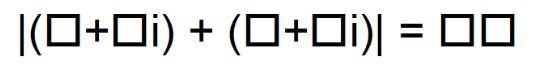# The Modulus Of A Complex Number

Directions: Using the digits 0 to 9 at most one time each, place a digit in each box to find an odd modulus, an even modulus, and the smallest possible modulus.### Hint

Consider Pythagorean Triples.

|(3+8i) + (6+4i)| = 15
|(7+8i) + (9+4i)| = 20
|(1+2i) + (5+6i)| = 10

Source: Mark Ward

### Check Also

Directions: Using the digits 1 to 9 at most one time each, place a digit …

1.yu neede to mack tis alot eesier way t0 harrd

•yeh whay to hahrd

2.David Campbell

|(5 + 4i) + (0 + 8i)| = 13.

Consider removing the third solution as the number 1 is used twice.